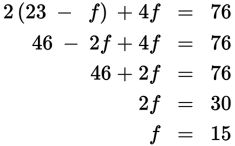# SAT Math Multiple Choice Question 809: Answer and Explanation

### Test Information

Question: 809

14. In a college art class, 76 students are painting a mural on one wall of the campusamphitheater. The wall has been divided into 23 sections, and each section will bepainted by a group of either 2 or 4 students. How many more sections will be paintedby a group of 4 students than by a group of 2 students?

• A. 6
• B. 7
• C. 8
• D. 9

Explanation:

B

Difficulty: Hard

Category: Heart of Algebra / Systems of Linear Equations

Strategic Advice: Assign variables to the unknowns. Then translate from English into math to create a system of equations-one equation for the number of sections and one equation for the number of students.

Getting to the Answer: Let t represent the number of sections of the wall that will be painted by a group of 2 students and f represent the number of sections that will be painted by a group of 4 students. Because there are 23 sections that will be painted by 76 students, it follows that t + f = 23 and 2t + 4f = 76. Solve this system of equations using substitution: Solve the first equation for t to get t = 23 - f. Then substitute this expression into the second equation for t and solve for f:The number of groups of 4 students is 15, which means the number of groups of 2 students is t = 23 - 15 = 8. Therefore, 15 - 8 = 7 more of the sections will be painted by a group of 4 students than by a group of 2 students, making (B) correct.